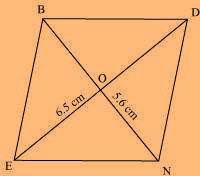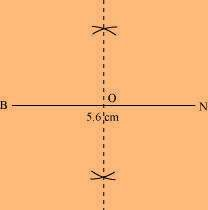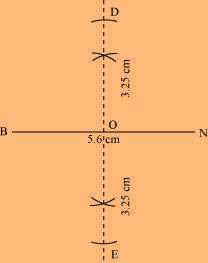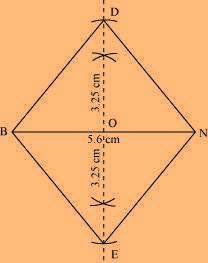# Construct the following quadrilateral: Rhombus BEND. BN = 5.6 cm DE = 6.5 cm

Solution

We know that the diagonals of a rhombus always bisect each other at 90º.

Let us predict that these are intersecting each other at point O in this rhombus. Hence, EO = OD = 3.25 cm

The rough sketch of the rhombus BEND can be drawn as per the steps given below(1) Draw a line segment BN of 5.6 cm and also draw its perpendicular bisector. Let it intersect the line segment BN at point O.(2) Considering O as centre, draw arcs of 3.25 cm radius to intersect the perpendicular bisector at point D and E.(3) Join points D and E to points B and N.